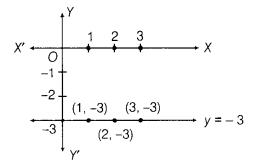# Draw the graph of the equation represented by a straight line

Draw the graph of the equation represented by a straight line which is parallel to the X-axis and at a distance of 3 units below it.

Any straight line parallel to X-axis and below to it, i.e. in negative direction of X-axis, is given by y = -k, . where k is the distance of the line from the X-axis. Here, k = 3, therefore the equation of the line is y = - 3.
To draw the graph of this equation, plot the points (1, -3), (2, - 3)and (3,-3) and by joining them to get a line of the required graph.